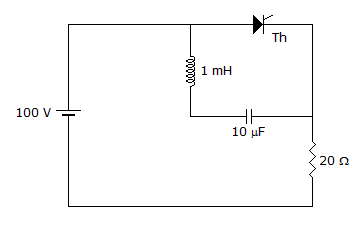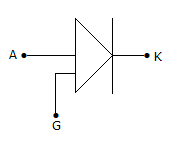Courses

Test: Power Electronics - 5

25 Questions MCQ Test Power Electronics | Test: Power Electronics - 5

Description
Attempt Test: Power Electronics - 5 | 25 questions in 25 minutes | Mock test for Electrical Engineering (EE) preparation | Free important questions MCQ to study Power Electronics for Electrical Engineering (EE) Exam | Download free PDF with solutions
QUESTION: 1

Solution:
QUESTION: 2

Solution:
QUESTION: 3

In a parallel inverter

Solution:
QUESTION: 4

A step up chopper can give an output voltage

Solution:
QUESTION: 5

The specified maximum junction temperature of thyristors is about

Solution:
QUESTION: 6

A bridge type single phase cycloconverter changes the frequency f to f/3. Then one half wave of output contains

Solution:
QUESTION: 7

A half bridge inverter

Solution:
QUESTION: 8

A 3 phase series inverter has

Solution:
QUESTION: 9

In figure, thyristor Th is in off state. When thyristor is turned on, the peak thyristor current can beSolution:
QUESTION: 10

HVDC systems are preferable for only long distances.

Solution:
QUESTION: 11

Surge current rating of an SCR is

Solution:
QUESTION: 12

Which of the following devices has metal-silicon junction?

Solution:
QUESTION: 13

In a single phase full wave converter (M-2 connection) feeding on R-L load the input voltage is v = Vm sin ωt. The expression for rms output voltage is

Solution:
QUESTION: 14

A dc chopper is feeding an RLE load. The condition for maximum ripple is

Solution:
QUESTION: 15

In a series inverter

Solution:
QUESTION: 16

The total number of leads in SUS, SBS and SCS respectively are

Solution:
QUESTION: 17

Which of these devices can conduct in both directions?

Solution:
QUESTION: 18

Assertion (A): A half wave controlled rectifier has poorer efficiency and higher ripple factor than a full wave controlled rectifier.

Reason (R): The use of a freewheeling diode in half wave controlled rectifier circuit improves the waveform of load current and circuit power factor.

Solution:
QUESTION: 19

A 3 phase dual converter consists of

Solution:
QUESTION: 20

Which inverter circuit uses coupled inductors?

Solution:
QUESTION: 21

When an SCR is in forward blocking mode, the applied voltage

Solution:
QUESTION: 22

In sinusoidal PWM for inverters the width of different pulses are not the same.

Solution:
QUESTION: 23

A class D chopper

Solution:
QUESTION: 24

The symbol in figure is forSolution:
QUESTION: 25

The efficiency of a chopper circuit is about

Solution:Use Code STAYHOME200 and get INR 200 additional OFF Use Coupon Code

Track your progress, build streaks, highlight & save important lessons and more!

Similar ContentRelated tests